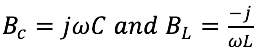|
|### What is the Inverse of Impedance, Reactance, and Resistance?

March 22, 2023

The inverse of impedance is admittance. Traditionally, impedance is expressed as R+jX, where R is resistance and X is reactance, both in units of ohms. Admittance is the inverse of impedance and is expressed as G+jB, where G is conductance and B is susceptance. Conductance is the inverse of resistance, and B is the inverse of reactance. Both have units of mhos.

Capacitive and inductive susceptance are given by: